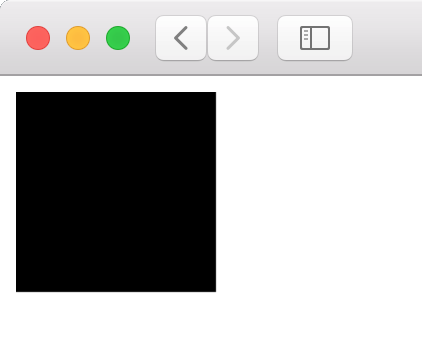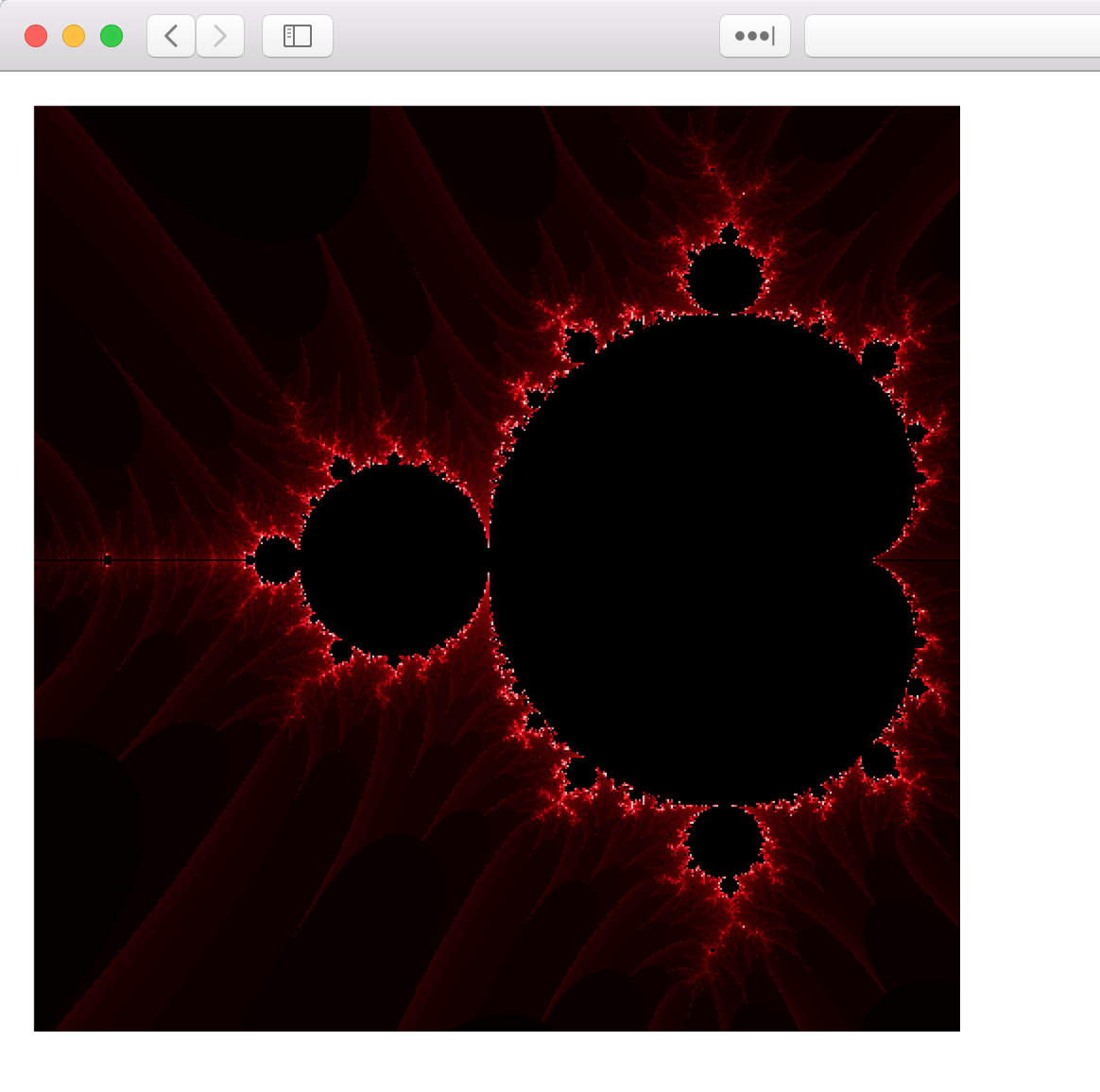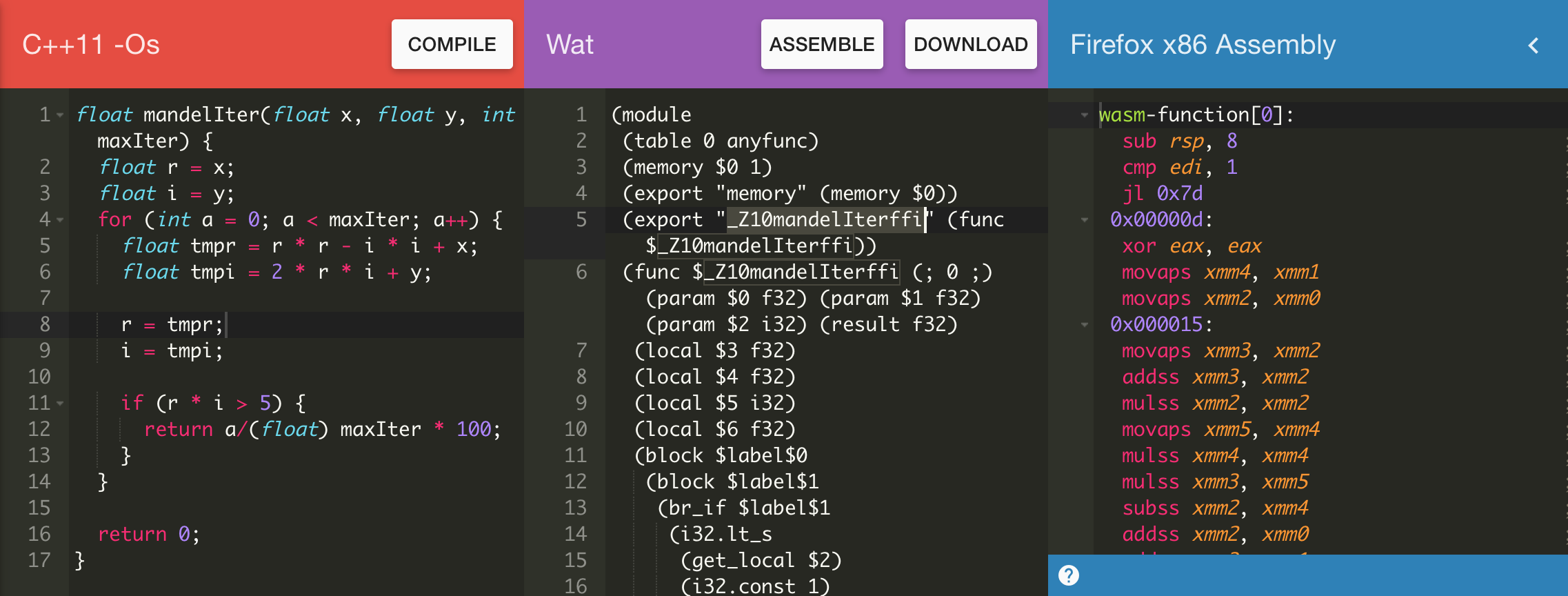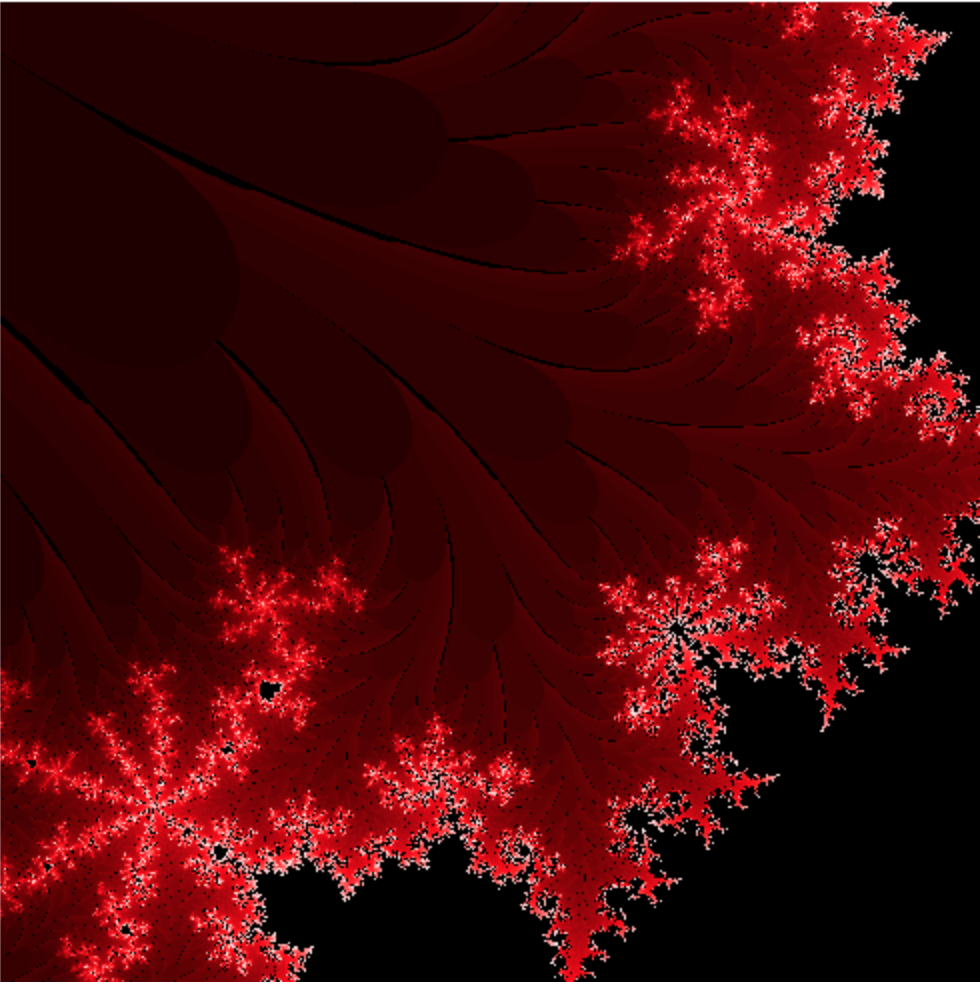8 mins

React JavaScript WASM WebAssembly

Edit

# Using WebAssembly with React

WebAssembly (WASM) is a binary format for the executable code in the browsers. In this article, we will create a simple web application using React library, write and compile to WASM a part of our JavaScript code and after that link it to the application.

We need a minimal application with a React library. I don’t describe how to create it from scratch, you can read about it in the article The minimal React + Webpack 4 + Babel Setup. The application in this repository is enough for our needs.

## Preparing

To start using the minimal React application we can clone the repository:

``````\$ git clone git@github.com:rwieruch/minimal-react-webpack-babel-setup.git wasm_react
``````

Now we can install all dependencies and start the server:

``````\$ cd wasm_react
\$ yarn install
\$ yarn start
``````

After that you can go to http://localhost:8080 and check if it works.

## Create canvas component

The next thing we should do is to create a new React component with canvas and add the function to drawing.

For our new component we can create the new file:

``````\$ touch src/canvas.js
``````

And put in it this code:

``````// src/canvas.js
import React, {Component} from "react";

class Canvas extends Component {

componentDidMount() {
let canvas = this.refs.canvas.getContext('2d');
canvas.fillRect(0, 0, 100, 100);
}

render() {
return (
<canvas ref="canvas" width={this.props.width} height={this.props.height}/>
)
}
}

export default Canvas;
``````

This component creates canvas using parameters from `props` and after that you should see a black rectangle in canvas.

For rendering the new component we can add it to `src/.js`:

``````// src/.js
import React from 'react';
import ReactDOM from 'react-dom';

import Canvas from './canvas';

const title = 'My Minimal React Webpack Babel Setup';

ReactDOM.render(
<Canvas height={500} width={500} />,
document.getElementById('app')
);

module.hot.accept();
``````

Now you can go to a browser and check if you can see a black rectangle:## Drawing fractals

The next thing what we will draw is incredibly beautiful Mandelbrot sets. First, we will implement it using JavaScript and after that we will reimplement it in WebAssembly. More theoretical background about that you can find in this article. I have just got the main function from this article.

Now we can add the `mandelIter` function to our Canvas component:

``````// scr/canvas.js
class Canvas extends Component {

//.....

mandelIter(x, y, maxIter) {
let r = x;
let i = y;
for (let a = 0; a < maxIter; a++) {
let tmpr = r * r - i * i + x;
let tmpi = 2 * r * i + y;

r = tmpr;
i = tmpi;

if (r * i > 5) {
return a/maxIter * 100;
}
}

return 0;
}

//.....
``````

After that, we can add to `componentDidMount` two loops to iterate over the all pixels in the canvas.

The updated function:

``````// src/canvas.js
componentDidMount() {
let canvas = this.refs.canvas.getContext('2d');
let mag = 200;
let panX = 2;
let panY = 1.25;
let maxIter = 100;

for (let x = 10; x < this.props.height; x++)  {
for (let y = 10; y < this.props.width; y++)  {
let m = this.mandelIter(x/mag - panX, y/mag - panY, maxIter);
canvas.fillStyle = (m === 0) ? '#000' : 'hsl(0, 100%, ' + m + '%)';
canvas.fillRect(x, y, 1,1);
}
}
}
``````

After this change you can see the Mandelbrot set on the page:It looks great, doesn’t it?

## Implementing in WebAssembly

Now we can implement a function `mandelIter` in WebAssembly. We can do it by using C++, Rust or Go. But here we will use C++ and an online compiler WebAssembly Explorer:

The function `mandelIter` implemented in C++:

``````float mandelIter(float x, float y, int maxIter) {
float r = x;
float i = y;
for (int a = 0; a < maxIter; a++) {
float tmpr = r * r - i * i + x;
float tmpi = 2 * r * i + y;

r = tmpr;
i = tmpi;

if (r * i > 5) {
return a/(float) maxIter * 100;
}
}

return 0;
}
``````

Our function after the compilation has some strange name: `_Z10mandelIterffi`. We will use this name in our JavaScript code.After compiling, we can download and put the file in `src` folder. I have named it `fractal.wasm`.

For using wasm in React you just need to add import to `Canvas`-component:

``````// src/canvas.js
import React, {Component} from "react";

const wasm = import("./fractal.wasm");

class Canvas extends Component {
``````

The next step is updating the `componentDidMount` function:

``````// src/canvas.js

componentDidMount() {
wasm.then(wasm => {
const mandelIterWASM = wasm._Z10mandelIterffi;
let canvas = this.refs.canvas.getContext('2d');
let mag = 200;
let panX = 2;
let panY = 1.25;
let maxIter = 100;

for (let x = 10; x < this.props.height; x++)  {
for (let y = 10; y < this.props.width; y++)  {
// let m = this.mandelIter(x/mag - panX, y/mag - panY, maxIter);
let m = mandelIterWASM(x/mag - panX, y/mag - panY, maxIter);
canvas.fillStyle = (m === 0) ? '#000' : 'hsl(0, 100%, ' + m + '%)';
canvas.fillRect(x, y, 1,1);
}
}
});
}

``````

Now for drawing on canvas we are using the function implemented in WebAssembly.

You can manipulate variables `mag`, `panX` and `panY` to create another form of fractals:All code you can find in my repository.